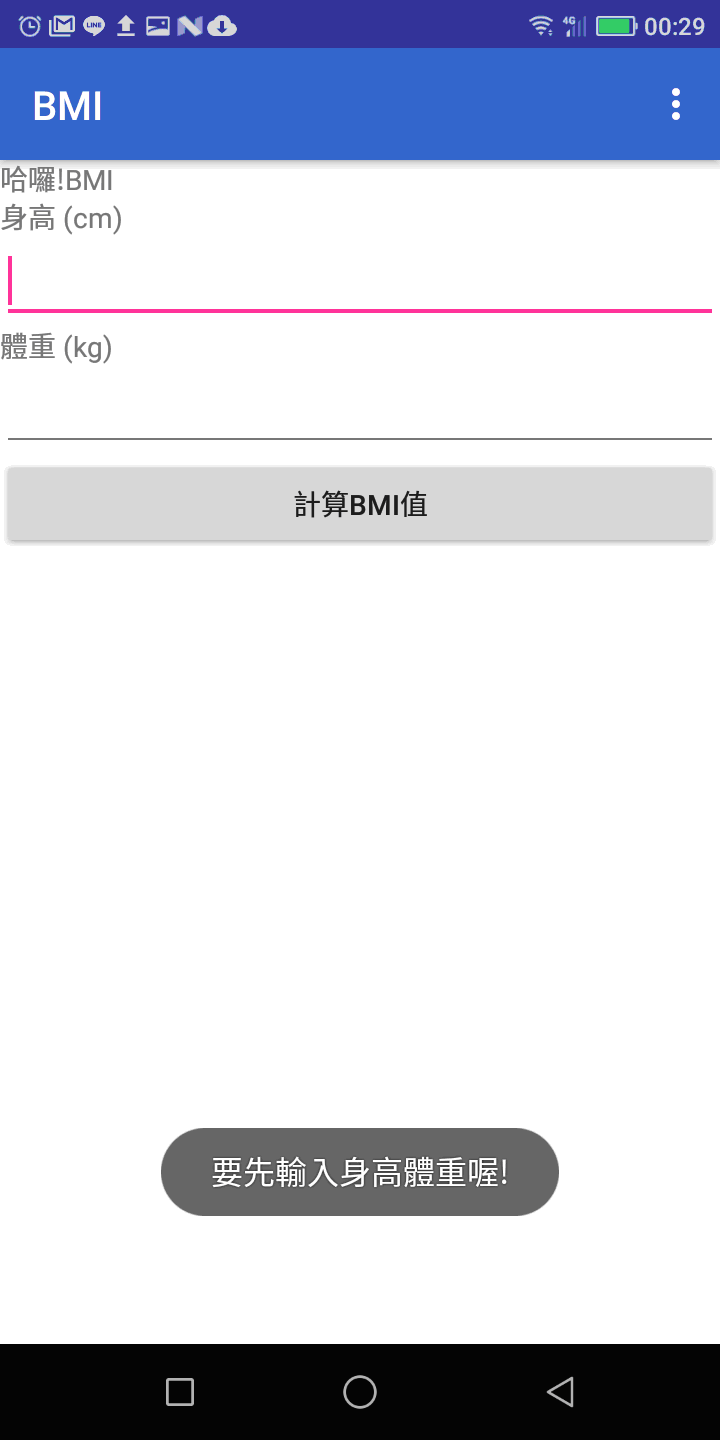#2019 iT 邦幫忙鐵人賽DAY 23
2
Software Development

## [Day 22] 我的第一個Android程式 - BMI程式設計(十三) 錯誤處理

MainActivity.java

``````private OnClickListener calcBMI = new OnClickListener() {
@Override
public void onClick(View v) {
try
{
DecimalFormat nf = new DecimalFormat("0.00");
//身高
double height = Double.parseDouble(num_height.getText().toString())/100;
//體重
double weight = Double.parseDouble(num_weight.getText().toString());
//計算出BMI值
double BMI = weight / (height*height);

//結果
show_result.setText(getText(R.string.bmi_result) + nf.format(BMI));

//建議
if(BMI > 25) //太重了
show_suggest.setText(R.string.advice_heavy);
else if(BMI < 20) //太輕了
show_suggest.setText(R.string.advice_light);
else //剛剛好
show_suggest.setText(R.string.advice_average);
}
catch(Exception obj)
{
Toast.makeText(MainActivity.this, "要先輸入身高體重喔!", Toast.LENGTH_SHORT).show();
}
}
};
``````### 1 則留言

0

{
Toast.makeText(MainActivity.this, "要先輸入身高體重喔!", Toast.LENGTH_SHORT).show();
}catch後面為什麼換行了, 所以他有錯誤訊息嗎?